# Accounting for autocorrelation in R

by Mark R Payne
DTU-Aqua, Charlottenlund, Denmark
http://www.staff.dtu.dk/mpay

Wed Jan 13 11:11:07 2016

Spatial and temporal correlations between data points are a common feature of most datasets in Marine Science. However, accounting and correcting for these features is not necessarily straight forward. In this tutorial, I demonstrate how this can be done in some of the most simple cases.

## An example data set

To start with we need a simple data set to work with. We could generate our own, but lets follow the analysis of Zuur et al 2009, and the Hawaiian bird example. This data set can be downloaded from http://www.highstat.com/book2.htm

dat.raw <- read.table("Hawaii.txt",header=TRUE)
dat.all <- data.frame(year=dat.raw$Year,birds=sqrt(dat.raw$Moorhen.Kauai),rain=dat.raw$Rainfall) There are missing values in 1972, 1965 and 1957. To start with we will only look at the part of the time series that is complete, from from 1973 onwards. dat <- subset(dat.all,year>=1973) save(dat,file="Correlation_data.RData") ## Exercises • Plot the time series of data • Estimate the linear (time) trend in the data, ignoring the effect of covariates such as rainfall. • Is the trend significant? • Perform normal model diagnostics. Are the residuals normally distributed? How does the Tukey-Anscombe plot look? • Is there evidence of autocorrelation in the residuals? • Now estimate the linear (time) trend again, but this time accounting for AR1 autocorrelation. Is • Is the trend significant? • Perform normal model diagnostics. Are the residuals normally distributed? How does the Tukey-Anscombe plot look? • Is there evidence of autocorrelation in the residuals? • Which of the two is the “better” model? Why? ## Linear trend analysis First, plotting the data shows that we have got more or less the same thing as in Zuur’s book, just truncated. plot(dat$year,dat\$birds)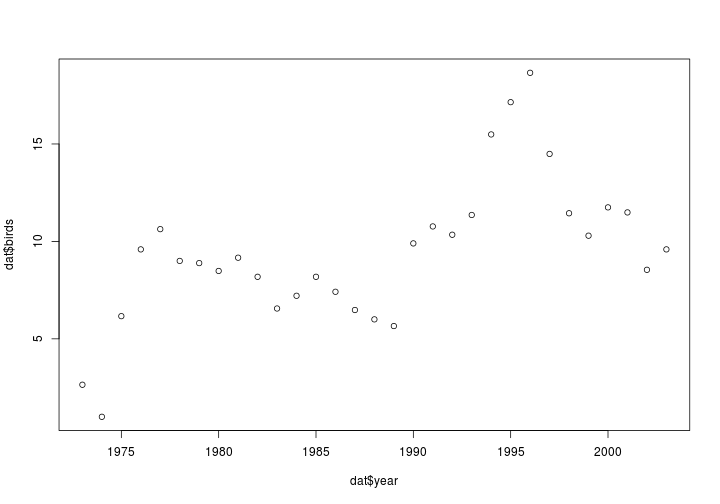Now lets fit a linear trend, ignorning any potential covariates

mdl <- lm(birds ~year,data=dat)
summary(mdl)
##
## Call:
## lm(formula = birds ~ year, data = dat)
##
## Residuals:
##     Min      1Q  Median      3Q     Max
## -5.0179 -1.7426 -0.0977  1.5436  7.2647
##
## Coefficients:
##               Estimate Std. Error t value Pr(>|t|)
## (Intercept) -476.01539  121.04255  -3.933 0.000480 ***
## year           0.24419    0.06089   4.011 0.000389 ***
## ---
## Signif. codes:  0 '***' 0.001 '**' 0.01 '*' 0.05 '.' 0.1 ' ' 1
##
## Residual standard error: 3.032 on 29 degrees of freedom
## Multiple R-squared:  0.3568, Adjusted R-squared:  0.3346
## F-statistic: 16.09 on 1 and 29 DF,  p-value: 0.0003887

which suggest a highly significant slope (p<0.001). How do the model diagnostics look?

par(mfrow=c(2,2))
plot(mdl)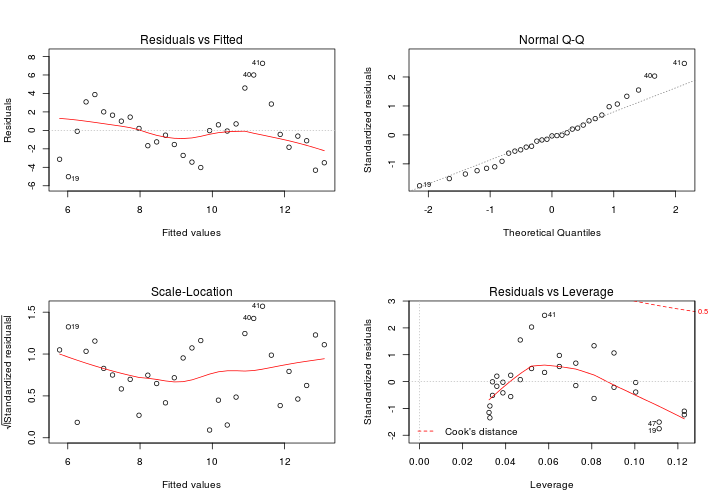The Tukey-Anscombe plot (top-left) suggests that the variance of the residuals is constant, as does the scale-location plot (bottom-left). The QQ plot looks ok-ish. There don’t appear to be any bad outliers (bottom-right) How does the time series of residuals look?

par(mfrow=c(1,1))
plot(residuals(mdl))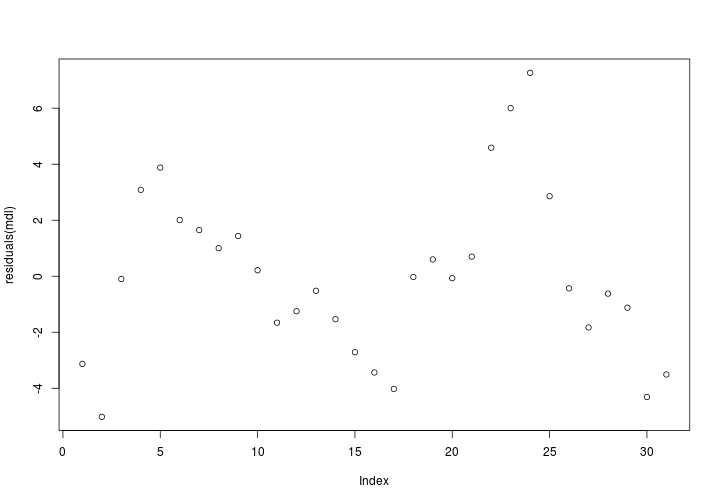Looks like there are some trends there. Doing the dot-to-dot makes it obvious

plot(residuals(mdl),type="b")
abline(h=0,lty=3)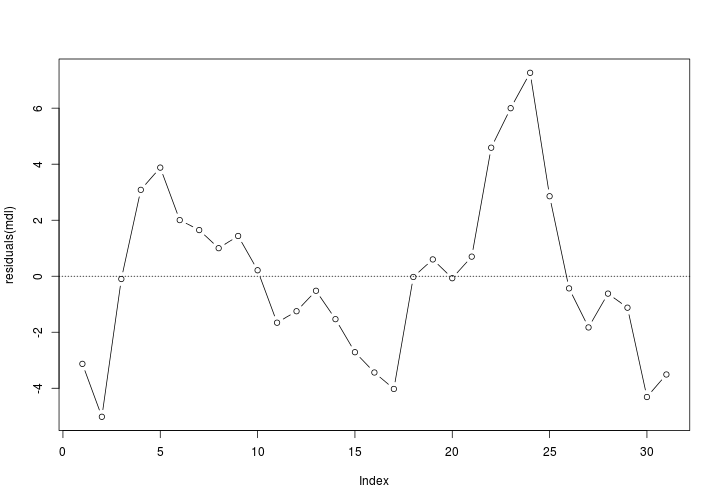So, lets make the autocorrelation plot

acf(residuals(mdl))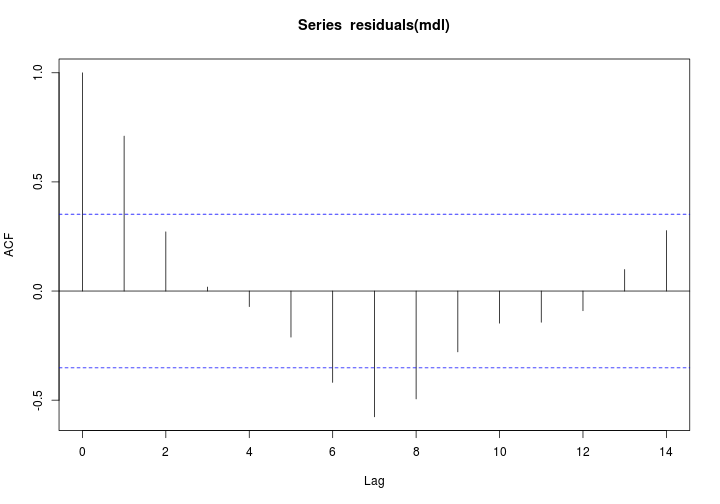Looks like we have some work to do! ## Linear trend analysis with autocorrelation So, lets account for that autocorrelation. We do this using the gls() function in the nlme package.

library(nlme)
mdl.ac <- gls(birds ~year, data=dat,
correlation = corAR1(form=~year),
na.action=na.omit)
summary(mdl.ac)
## Generalized least squares fit by REML
##   Model: birds ~ year
##   Data: dat
##        AIC      BIC    logLik
##   137.7397 143.2089 -64.86987
##
## Correlation Structure: AR(1)
##  Formula: ~year
##  Parameter estimate(s):
##       Phi
## 0.9132806
##
## Coefficients:
##                 Value Std.Error  t-value p-value
## (Intercept) -457.9346  455.1807 -1.00605  0.3227
## year           0.2344    0.2290  1.02386  0.3144
##
##  Correlation:
##      (Intr)
## year -1
##
## Standardized residuals:
##        Min         Q1        Med         Q3        Max
## -0.7504240 -0.0730259  0.2199919  0.5526805  1.7095733
##
## Residual standard error: 5.080289
## Degrees of freedom: 31 total; 29 residual

Which is a pretty different result - the trend is no-longer significant! As a side note, in this situation, the adding auto-correlation does not change the slope, but does change the associated p-value e.g.

coef(mdl)
##  (Intercept)         year
## -476.0153912    0.2441911
coef(mdl.ac)
## (Intercept)        year
## -457.934600    0.234421

Lets check the diagnostics. These have to be done by hand this time. First, the Tukey Anscombe

plot(fitted(mdl.ac),residuals(mdl.ac))
abline(h=0,lty=3)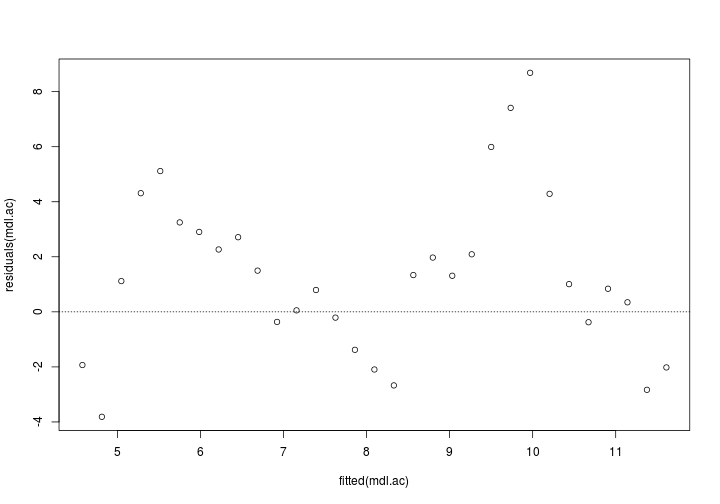Ok. And the qqplot

qqnorm(mdl.ac)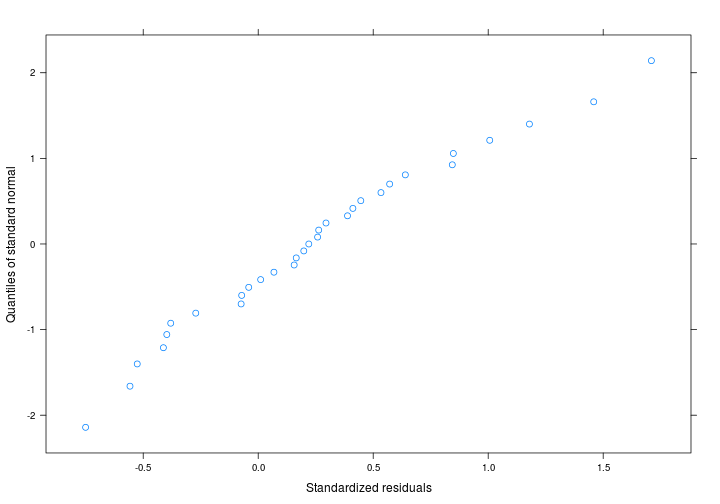And the acf of the residuals

acf(residuals(mdl.ac,type="p"))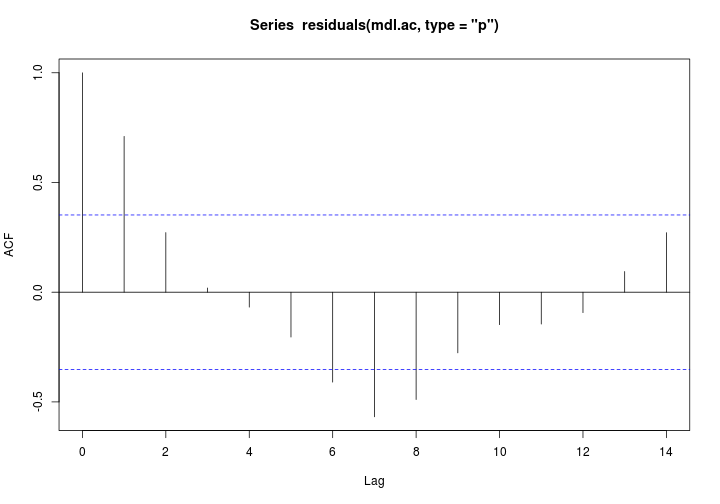## The “best” model?

How do we decide on the best model? By AIC of course!

library(MuMIn)
model.sel(mdl,mdl.ac)
## Model selection table
##        (Intrc)   year   family class   correlation na.action df logLik  AICc delta weight
## mdl.ac  -457.9 0.2344 gaussian   gls corAR1(~year)   na.omit  4 -64.87 139.3  0.00      1
## mdl     -476.0 0.2442 gaussian    lm                          3 -77.34 161.6 22.29      0
## Models ranked by AICc(x)

The autocorrelated version of the model is an easy winner.

 This work by Mark R Payne is licensed under a Creative Commons Attribution-NonCommercial-ShareAlike 3.0 Unported License. For details, see http://creativecommons.org/licenses/by-nc-sa/3.0/deed.en_US Basically, this means that you are free to “share” and “remix” for non-commerical purposes as you see fit, so long as you “attribute” me for my contribution. Derivatives can be distributed under the same or similar license. This work comes with ABSOLUTELY NO WARRANTY or support. *This work should also be considered as BEER-WARE. For details, see http://en.wikipedia.org/wiki/Beerware*